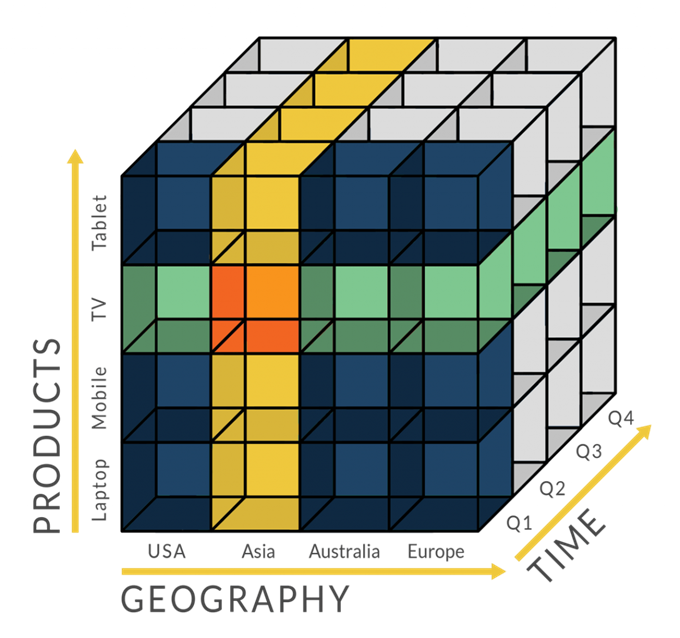# A/B测试所需样本量计算方法

A/B测试是我们在日常工作中经常使用的工具，A/B测试的核心就是：确定两个元素或版本（A和B）哪个版本更好，你需要同时实验两个版本。最后，选择最好的版本使用。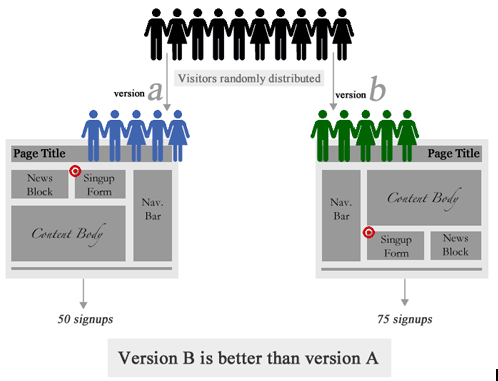A/B比较困难的点是确定在什么时候可以终止实验。当样本量不够时，监测的“转化率”变化数据可能是由于样本的不均匀导致的。  原则上实现的时间越长，样本量越大，实验的可靠性越高。但是实际过程中我们期望样本量越少越好，如果能提前知道实验结果，则可以快速的进行的版本切换，而不会浪费流量。这就涉及到如何计算实验的最小样本量。比较简单的是使用现成的工具：Evan’s Awesome A/B Tools

## 样本量计算器功能简介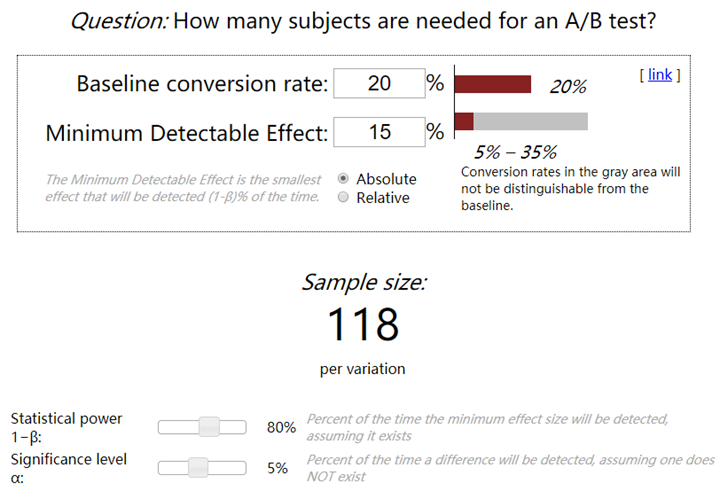• Baseline conversion rate（BCVR）：原始版本转化率
• Minimum Detectable Effect（MDE）：最小可探测效应（需要监测的转化率效果）
• Absolute：绝对比例，BCVR±MDE
• Relative：相对比例，BCVR*(1±MDE)
• Statistical power $1-\beta$：检验效能Power，也有人称之为把握度，一般选8(即II型错误$\beta=0.2$)或0.9，检验效能越大，需要的样本量越多。
• Significance level $\alpha$：检验水准，临床试验中，检验水准$\alpha$一般取05(双侧检验)或0.025(单侧检验)

## 样本量计算器原理说明

### 中心极限定理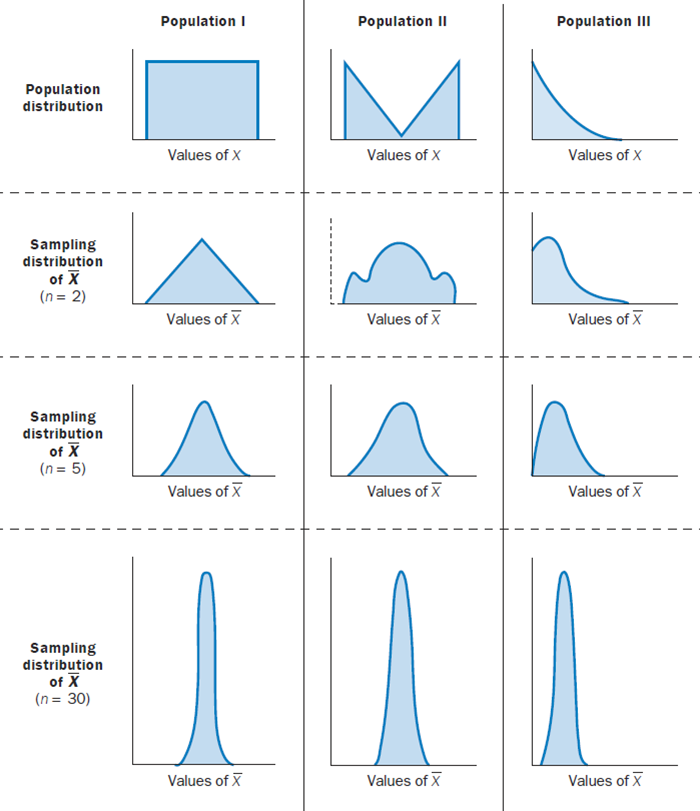### 假设检验

• 原假设 H0：我们反对的假设（样本与总体或样本与样本间的差异是由抽样误差引起的）
• 备择假设 H1：我们坚持的假设（样本与总体或样本与样本间存在本质差异）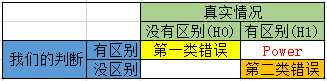• 第一类错误（Type I error）：实际没有区别，我们的判断是有区别。我们把第一类错误出现的概率用$\alpha$表示。这个$\alpha$，就是Significance Level。
• 第二类错误（Type II error）：实际有区别，我们的判断是没有区别。用$\beta$表示。根据条件概率的定义，可以计算出$\beta = 1 – power$。

• 第一类错误$\alpha$不超过5%。也就是说，Significance Level = 5%。
• 第二类错误$\beta$不超过20%。也就是说，Statistical Power = 1 – $\beta$ = 80%。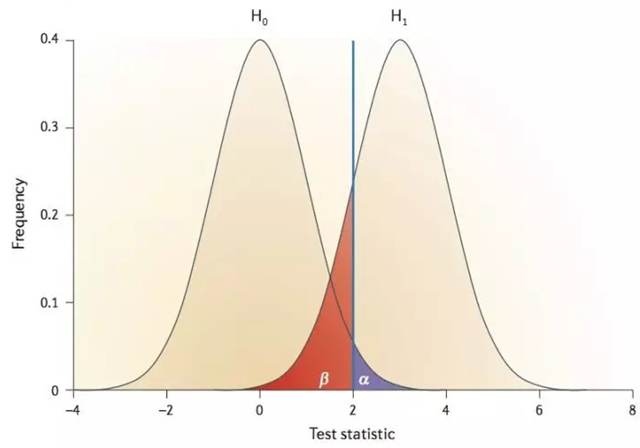### 样本量计算

$$n = \frac{\sigma ^2}{\Delta^2}(Z_{\frac{\alpha }{2}}+Z_{\beta })^2$$

• n是每组所需样本量，因为A/B测试一般至少2组，所以实验所需样本量为2n
• $\alpha$和$beta$分别称为第一类错误概率和第二类错误概率，一般分别取05和0.2
• Z为正态分布的分位数函数
• $\Delta$为两组数值的差异，如点击率1%到5%，那么$\Delta$就是0.5%
• $\sigma$为标准差，是数值波动性的衡量，$\sigma$越大表示数值波动越厉害。

$$n = \frac{(Z_{\frac{\alpha }{2}} \cdot \sqrt{2\cdot \frac{(p_1+p_2)}{2}\cdot (1-\frac{(p_1+p_2)}{2})}+Z_{\beta }\cdot \sqrt{p_1\cdot (1-p_1) + p_2\cdot (1-p_2)})^2}{|p_1-p_2|^2}$$

## 样本量计算的实现

Python实现：

from statsmodels.stats.power import zt_ind_solve_power
from statsmodels.stats.proportion import proportion_effectsize as es

zt_ind_solve_power(effect_size=es(prop1=0.30, prop2=0.305), alpha=0.05, power=0.8, alternative="two-sided")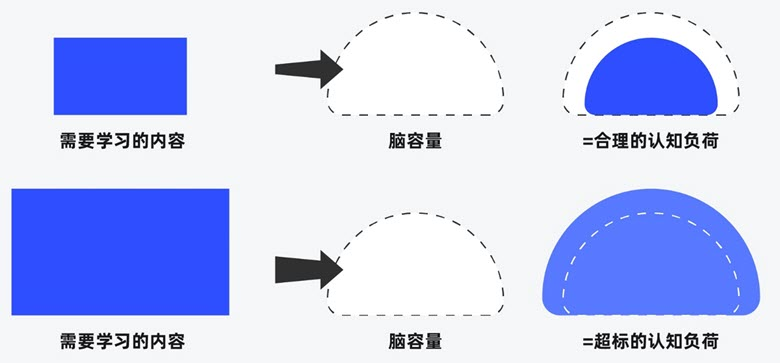##### 界面设计和游戏设计中的认知负荷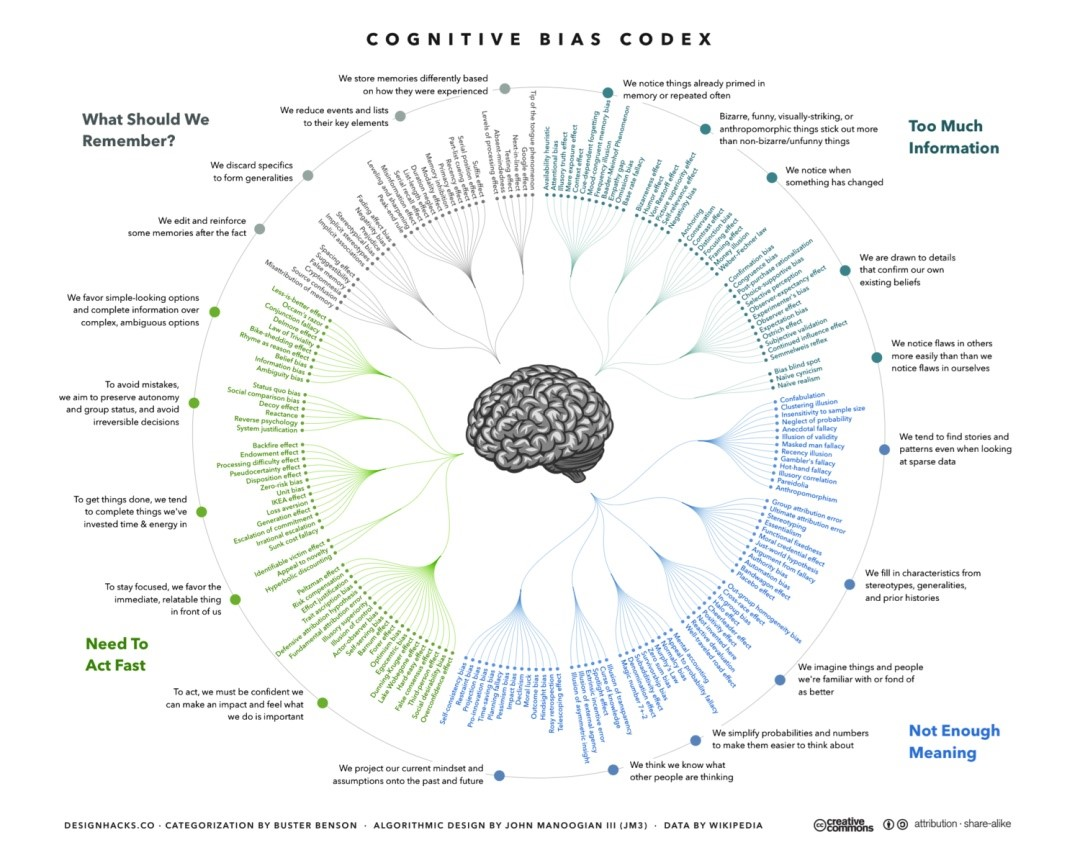##### 理解认知偏差